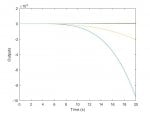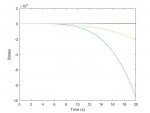# Matlab step input to a MIMO system

#### TrojanPoem

Script name: model.m

function dx = model(t, x)
g = 9.8; %Gravity
Iy = 0.5; %Interia
m = 0.2; %mass
A = [0 0 1 0 0 0;0 0 0 1 0 0; 0 0 0 0 0 -g;0 0 0 0 0 0; 0 0 0 0 0 0;0 0 0 0 1 0];
B = [0 0;0 0;0 0; 1/m 0;0 1/Iy; 0 0];

ft = unit_step(t,1);
ty = unit_step(t,1.5);
u = [ft; ty]; %The input

dx = A * x + B * u ;

%Step function used to find the current input to the system.
%For any other input define it here.
function u_val = unit_step(cur_time, step_time)

if cur_time > step_time
u_val = 1;
else
u_val = 0;
end

Script name: run_model.m

%simulation time from 0 sec to 20 sec

t_from = 0;

t_to = 20;

%initial states

x_o = 0;

z_o = 0;

u_o = 0;

w_o = 0;

q_o = 0;

theta_o = 0;

[t,x] = ode45(@model, [t_from t_to], [x_o z_o u_o w_o q_o theta_o]);

%The result is two vectors t, x , which contain the states and time steps

%To find the output, we could use the C matrix or just notice that the C is diagonal so all the states are outputs.

C = [1 0 0 0 0 0;0 1 0 0 0 0;0 0 1 0 0 0;0 0 0 1 0 0;0 0 0 0 1 0;0 0 0 0 0 1];

y =C*x'; %Just to demonstrate how to calculate it, but the plots will be the same.

plot(t,x);

xlabel('Time (s)');

ylabel('States');

figure;

plot(t,y);

xlabel('Time (s)');

ylabel('Outputs');

MATLAB figures:Note:

-I have chosen random numbers and step time to solve the problem with random initial states accordingly the plots does not seem to be correct as the system is unstable, but the method is assured.

-Another solution is to model it in simulink and apply the inputs which would be easier as it is done graphically.

#### Tran Thanh Dan

Script name: model.m

function dx = model(t, x)
g = 9.8; %Gravity
Iy = 0.5; %Interia
m = 0.2; %mass
A = [0 0 1 0 0 0;0 0 0 1 0 0; 0 0 0 0 0 -g;0 0 0 0 0 0; 0 0 0 0 0 0;0 0 0 0 1 0];
B = [0 0;0 0;0 0; 1/m 0;0 1/Iy; 0 0];

ft = unit_step(t,1);
ty = unit_step(t,1.5);
u = [ft; ty]; %The input

dx = A * x + B * u ;

%Step function used to find the current input to the system.
%For any other input define it here.
function u_val = unit_step(cur_time, step_time)

if cur_time > step_time
u_val = 1;
else
u_val = 0;
end

Script name: run_model.m

%simulation time from 0 sec to 20 sec

t_from = 0;

t_to = 20;

%initial states

x_o = 0;

z_o = 0;

u_o = 0;

w_o = 0;

q_o = 0;

theta_o = 0;

[t,x] = ode45(@model, [t_from t_to], [x_o z_o u_o w_o q_o theta_o]);

%The result is two vectors t, x , which contain the states and time steps

%To find the output, we could use the C matrix or just notice that the C is diagonal so all the states are outputs.

C = [1 0 0 0 0 0;0 1 0 0 0 0;0 0 1 0 0 0;0 0 0 1 0 0;0 0 0 0 1 0;0 0 0 0 0 1];

y =C*x'; %Just to demonstrate how to calculate it, but the plots will be the same.

plot(t,x);

xlabel('Time (s)');

ylabel('States');

figure;

plot(t,y);

xlabel('Time (s)');

ylabel('Outputs');

MATLAB figures:

View attachment 2364View attachment 2365

Note:

-I have chosen random numbers and step time to solve the problem with random initial states accordingly the plots does not seem to be correct as the system is unstable, but the method is assured.

-Another solution is to model it in simulink and apply the inputs which would be easier as it is done graphically.
Thanks mate, I got it done but with lsim command, I have the same plot so result wise both methods are good I guess. I'll definitely try out ode45 for my BLDC simulation.